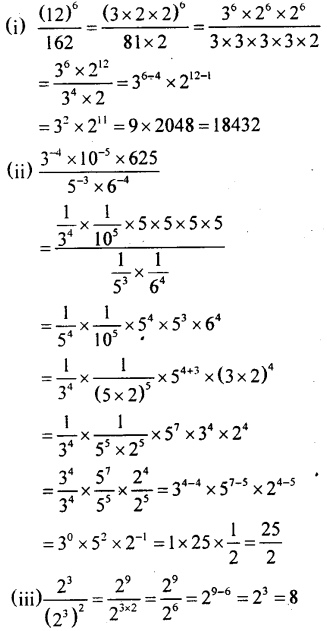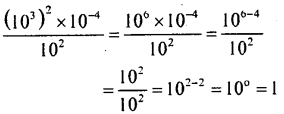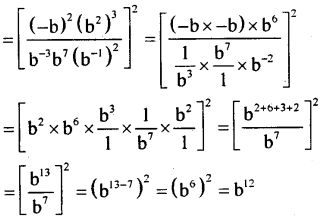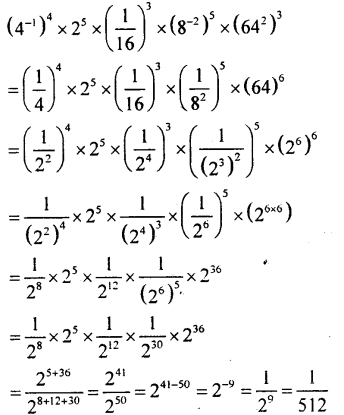# KSEEB Solutions for Class 8 Maths Chapter 10 Exponents Ex 10.7

In this chapter, we provide KSEEB SSLC Class 8 Maths Chapter 10 Exponents Ex 10.7 for English medium students, Which will very helpful for every student in their exams. Students can download the latest KSEEB SSLC Class 8 Maths Chapter 10 Exponents Ex 10.7 pdf, free KSEEB SSLC Class 8 Maths Chapter 10 Exponents Ex 10.7 pdf download. Now you will get step by step solution to each question.

### Karnataka State Syllabus Class 8 Maths Chapter 10 Exponents Ex 10.7

Question 1.
Use the laws of exponents and simplifyQuestion 2.
What is the value of (103)2×10−4102Question 3
Simplify:
[b−3⋅b7⋅(b−1)2(−b)2(b2)3]−2Question 4.
Find the value of each of the following expressions.

(a). (32)2 – (-2)3)– (-(5)2)2
= 34 – (- 2 x – 2 x – 2)2 – (-52 )2
= 34 – (-23)2 – 54
= 81 – 26 – 625
= 81- 64 – 625
= 81 – 689
= – 608

(b). ((0.6)2)– ((4.5)0)-2
= (0.6)2 x 0 – (4.5)0 x -2
= (0.6)0 – (4.5)0
= 1 – 1
= 0

(c).All Chapter KSEEB Solutions For Class 8 maths

—————————————————————————–

All Subject KSEEB Solutions For Class 8

*************************************************

I think you got complete solutions for this chapter. If You have any queries regarding this chapter, please comment on the below section our subject teacher will answer you. We tried our best to give complete solutions so you got good marks in your exam.

If these solutions have helped you, you can also share kseebsolutionsfor.com to your friends.

Best of Luck!!